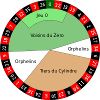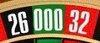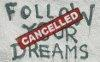okok0 3

- i bet in 1 column every time, how many times do i need to repeat my bet for my bet to win.
- i bet in 1 dozens every time, how many times do i need to repeat my bet for my bet to win.trizeroyouroul.com80 667

okok:

how many times do I need to repeat my bet for my bet to win.

First you must understand, that there is no difference in the mathematics of dozen and column.
It does not matter which 12 numbers you take for a bet, as long as your wheel has no tilt or something like this.

The probability for 12 numbers in a 37 numbers wheel to win is 12 / 37.
12 to 37 written decimal is 0.324324324... which means 32.43 % nearly 1 / 3, in every spin.
For two spins it's 12/37 · 12/37 = 0.105186267.
For n spins it's 12/37 ^ n (read 12/37 exponent n).

If you prefer to have integer numbers, counting such amounts, than you can build your roulette strategy here.
If you checked, that everything works as intended, then load a long test configuration, to run over hundreds of thousands or millions of coups.
You will find the count of bets in the statistics then. example test statisticstrizeroyouroul.com80 667

trizero:

For two spins it's 12/37 · 12/37 = 0.105186267.

I forgot to explain what this means.
0.105 is the probability to bet the winning dozen, column or any 12 numbers in two spins.
This means that you will succeed in 1 of 10 tries statistically.

Sounds complicated?
That's the reason I developed the roulette simulator for strategies, which makes those mathematics superfluous.banksy12 37

Thank you for saving us from the mathematical struggles of roulette strategies!
I can easily understand the test results of the simulator instead of that strange formulas.okok0 3

trizero:

Sounds complicated?
That's the reason I developed the roulette simulator for strategies, which makes those mathematics superfluous.

Thanks you.
From my analyse, i am see two column or two dozen he is repeat 15 time later he is go for the next
exemple :
column 3 and column 2 , repeat 15 time later he is out , and you see column1
the same for dozen
dozen 3 + dozen 2 later he is out , and you see dozen 1